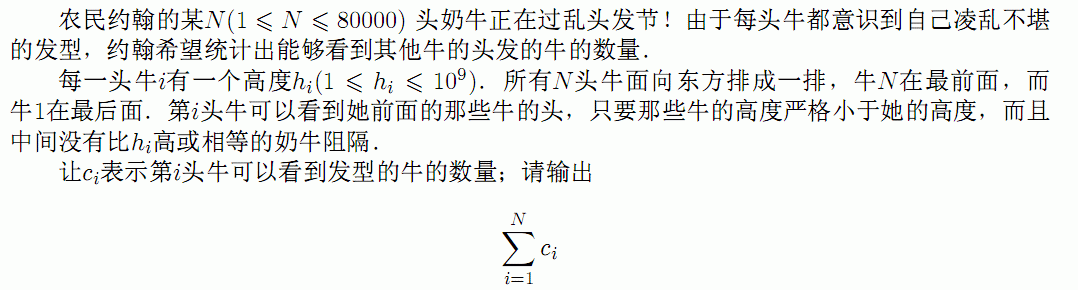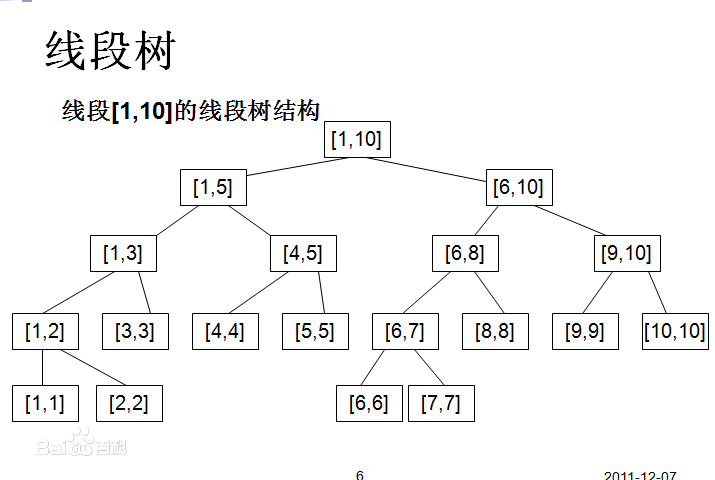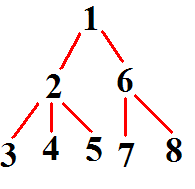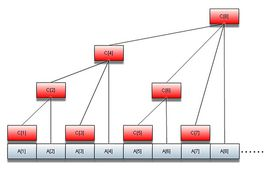# 单调栈、单调队列、线段树、LCA、二维树状数组、Bitset讲解

## 一、单调栈

1 6 4 8 9 2 3

### 例题：

bzoj1660#include<bits\stdc++.h>
using namespace std;
int Stack;
int top=0;
int main()
{
int n;
scanf("%d",&n);
long long ans=0;
for(int i=1;i<=n;i++)
{
int x;
scanf("%d",&x);
while(top && Stack[top]<=x) top--;
ans+=top;
Stack[++top]=x;
}
cout<<ans<<endl;
return 0;
}

## 二、单调队列

### 例题

#include<cstdio>
#include<cstdlib>
#include<ctime>
#include<cmath>
#include<iostream>
#include<iomanip>
#include<cstring>
#include<string>
#include<algorithm>
using namespace std;
int F;
int val;
int q;
int s=1,t=1;
int main()
{
int n,m;
scanf("%d%d",&n,&m);
for(int i=1;i<=n;i++) scanf("%d",&val[i]);
val[++n]=0;
q[t++]=0;
for(int i=1;i<=n;i++)
{
if(i-q[s]>m) s++;
F[i]=F[q[s]]+val[i];
while(t-s && F[i]<F[q[t-1]]) t--;
q[t++]=i;
}
cout<<F[n]<<endl;
return 0;
}

## 三、线段树

### 1.什么是线段树### 3.模板例题

#include<bits/stdc++.h>
using namespace std;
struct Seg_tree
{
int l,r,maxx;
}a;
int val;
void make_tree(int o,int l,int r)
{
a[o].l=l;
a[o].r=r;
if(l==r)
{
a[o].maxx=val[l];
return;
}
int mid=l+r>>1;
make_tree(2*o,l,mid);
make_tree(2*o+1,mid+1,r);
a[o].maxx=max(a[2*o].maxx,a[2*o+1].maxx);
}
int query(int o,int l,int r)
{
if(a[o].r<l || a[o].l>r) return -2147483647;
if(a[o].l>=l && a[o].r<=r) return a[o].maxx;
return max(query(2*o,l,r),query(2*o+1,l,r));
}

### 7.线段树分治

（这个我可能写的不清楚，听我讲吧QAQ

## 四、LCA

### 1.前言

LCA是算法竞赛中树上问题很重要的部分，掌握LCA基本上算是学会树上问题的第一步

### 2.LCA的主要算法

#### (1).倍增法

int deep;
int fa;
void dfs(int u,int fro)
{
deep[u]=deep[fro]+1;
fa[u]=fro;
for(int o=fir[u];o;o=nex[o]) if(a[o].r!=fro) dfs(a[o].r,u);
}
void init()
{
dfs(1,0);
for(int i=1;i<=17;i++)
for(int j=1;j<=200000;j++) fa[j][i]=fa[fa[j][i-1]][i-1];
}
int lca(int x,int y)
{
if(deep[x]<deep[y]) swap(x,y);
for(int i=17;i>=0;i--) if(deep[fa[x][i]]>=deep[y]) x=fa[x][i];
if(x==y) return x;
for(int i=17;i>=0;i--) if(fa[x][i]!=fa[y][i]) x=fa[x][i],y=fa[y][i];
return fa[x];
}

#### (2).ST表### 3.总结

LCA的算法很多，但我觉得这三种就已经够用了，倍增的优势是方便实现，ST表适合处理询问LCA次数特别多的题目，而树剖则是一个常数很小1的算法，适合询问LCA次数较少的题目，大家具体问题具体分析。

### 例题

NOIP2013货车运输
https://www.luogu.org/problem/show?pid=1967

## 五、二维树状数组

### 1.树状数组### 2.如何理解二维数据结构

（这个没有办法用文字来写，我现场讲）

### 3.二维树状数组和一维树状数组的区别

//在点x,y上加一个v
{
for(int i=x;i<=n;i+=i&(-i))
for(int j=y;j<=n;j+=j&(-j))
c[i][j]+=v;
}
//查询以（1,1）为左下角，（x,y）为右上角的子矩形的权值和
int get_val(int x,int y)
{
int re=0;
for(int i=x;i;i-=i&(-i))
for(int j=y;j;j-=j&(-j))
re+=c[i][j]
return re;
}

## 六、Bitset

（前天YihAN_Z讲STL的时候忘了讲了，今天我来填上这个坑

Bitset怎么用呢，请大家打开今天下发的C-free，然后听我讲。

### 例题

BZOJ3687

04-127396
11-1510
04-032万+
11-22284
08-111970
02-0872
08-19286
01-25100
07-251822
05-11128
07-17796
04-26206
08-131042
07-31111
08-2172
08-0273
11-051082
08-24406
02-13156
04-27553

### “相关推荐”对你有帮助么？

•非常没帮助
•没帮助
•一般
•有帮助
•非常有帮助被折叠的  条评论 为什么被折叠?到【灌水乐园】发言LZJ209

¥2 ¥4 ¥6 ¥10 ¥20余额支付 (余额：-- )扫码支付获取中扫码支付点击重新获取扫码支付1.余额是钱包充值的虚拟货币，按照1:1的比例进行支付金额的抵扣。
2.余额无法直接购买下载，可以购买VIP、C币套餐、付费专栏及课程。余额充值